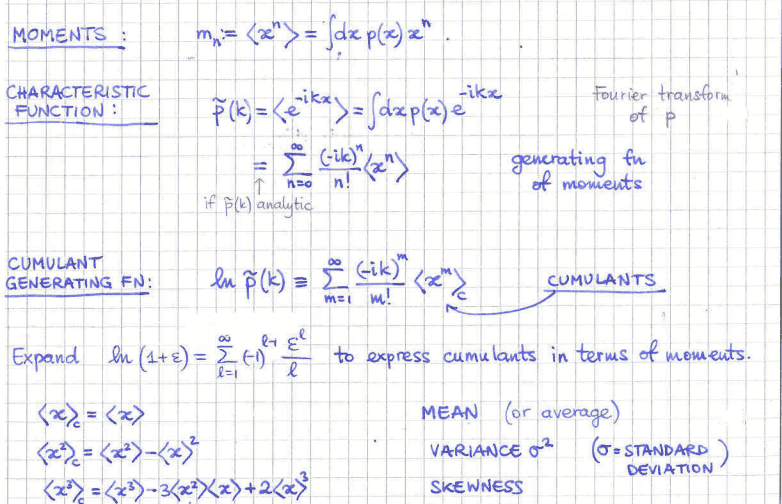# Statistical Mechanics- moments/cumulants, log expansion

binbagsss

## Homework Statement

Using log taylor expansion to express cumulants in terms of moments

I have worked through the expansion- ##log(1+\epsilon)= ...## see thumbnail- and that's ok; my question is why does the expansion hold, i.e. all i can see is it must be that ##k## is small- how is this defined as so?

In all my studies on fourier transforms, I don't ever recall talking about the size of the transform variable being small- (minus the exception of perhaps a boundary condition, and then k is a function of L and then as L gets large k is small) but why here would we require k small, and why isn't this mentioned?

## Homework Equations

my lectures notes here:as above

Homework Helper
Dearly Missed

## Homework Statement

Using log taylor expansion to express cumulants in terms of moments

I have worked through the expansion- ##log(1+\epsilon)= ...## see thumbnail- and that's ok; my question is why does the expansion hold, i.e. all i can see is it must be that ##k## is small- how is this defined as so?

In all my studies on fourier transforms, I don't ever recall talking about the size of the transform variable being small- (minus the exception of perhaps a boundary condition, and then k is a function of L and then as L gets large k is small) but why here would we require k small, and why isn't this mentioned?

## Homework Equations

my lectures notes here:View attachment 232140

## The Attempt at a Solution

as above

The expansion
$$\ln(1+r) = \sum_{k=1}^{\infty} (-1)^{k-1} \frac{r^k}{k} \hspace{4ex}(1)$$ holds only for ##|r| < 1.## Thus, if you write
$$\rho(k) = 1 + \underbrace{\sum_{n=1}^{\infty} \frac{(-ik)^n}{n!} \langle x^n \rangle}_{=\;r}$$
you need ##|k|## small enough to ensure ##|r| < 1## in order to be able to apply expansion (1) to ##\tilde{\rho}(k) = \ln \rho(k).## (Since ##r = r(k)## is continuous in ##k## and vanishes when ##k=0##, there will be a ##k##-interval surrounding ##0## that ensures ##|r(k)| < 1## whenever ##k## lies in that interval.)

binbagsss
The expansion
$$\ln(1+r) = \sum_{k=1}^{\infty} (-1)^{k-1} \frac{r^k}{k} \hspace{4ex}(1)$$ holds only for ##|r| < 1.## Thus, if you write
$$\rho(k) = 1 + \underbrace{\sum_{n=1}^{\infty} \frac{(-ik)^n}{n!} \langle x^n \rangle}_{=\;r}$$
you need ##|k|## small enough to ensure ##|r| < 1## in order to be able to apply expansion (1) to ##\tilde{\rho}(k) = \ln \rho(k).## (Since ##r = r(k)## is continuous in ##k## and vanishes when ##k=0##, there will be a ##k##-interval surrounding ##0## that ensures ##|r(k)| < 1## whenever ##k## lies in that interval.)

Thank you for your reply, and where is it assumed in the notes that k is small enough ? Is this a common sort of assumption with Fourier transforms or not ? Ta.

Gold Member
any time you see a series with a constraint like ##\big \vert r \big \vert \lt 1## you should immediately ponder the geometric series as a building block.
- - - -
It looks like your author if defining the cumulant as the log of the characteristic function... I've always seen it the other way: cumulant is log of MGF.

It's worth contemplating the differences here.